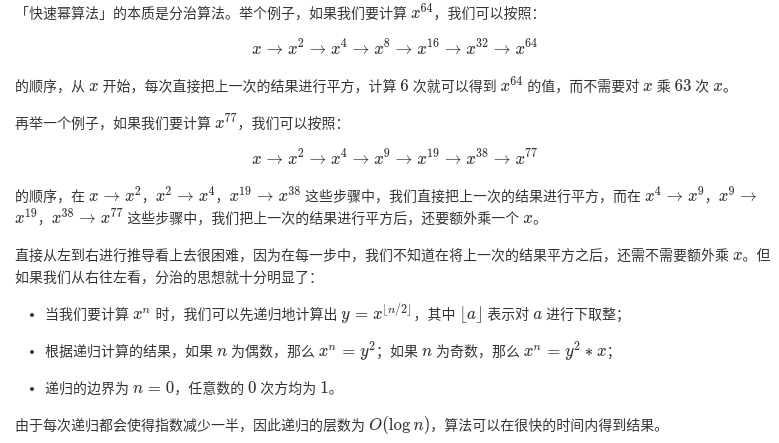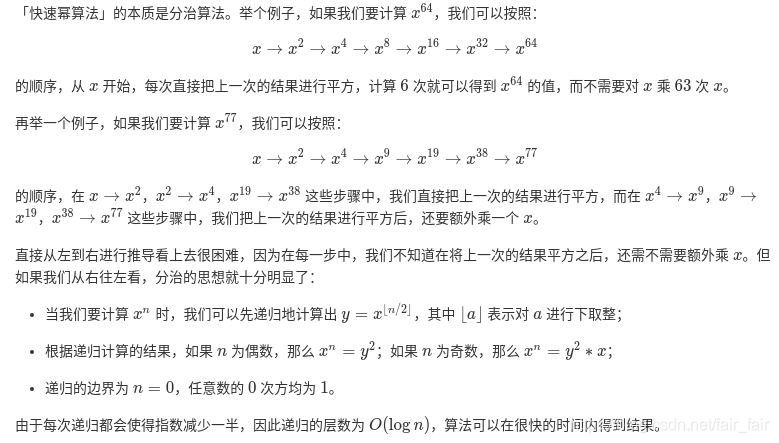• 以下是 math 模块 pow() 方法语法:importmathmath.pow(x,y)内置 pow() 方法pow(x,y[,z])函数是计算xy次方，如果z在存在，则再对结果进行取模，其结果等效于pow(x,y) %z注意：pow() 通过内置方法直接调用，...pow() 方法返回 xy(x的y次方) 的值。以下是 math 模块 pow() 方法的语法:import mathmath.pow( x, y )内置的 pow() 方法pow(x, y[, z])函数是计算x的y次方，如果z在存在，则再对结果进行取模，其结果等效于pow(x,y) %z注意：pow() 通过内置的方法直接调用，内置方法会把参数作为整型，而 math 模块则会把参数转换为 float。参数x – 数值表达式。y – 数值表达式。z – 数值表达式。返回值返回 xy(x的y次方) 的值。以下展示了使用 pow() 方法的实例：实例#!/usr/bin/python# -*- coding: UTF-8 -*-import math   # 导入 math 模块print "math.pow(100, 2) : ", math.pow(100, 2)# 使用内置，查看输出结果区别print "pow(100, 2) : ", pow(100, 2)print "math.pow(100, -2) : ", math.pow(100, -2)print "math.pow(2, 4) : ", math.pow(2, 4)print "math.pow(3, 0) : ", math.pow(3, 0)以上实例运行后输出结果为：math.pow(100, 2) :  10000.0pow(100, 2) :  10000math.pow(100, -2) :  0.0001math.pow(2, 4) :  16.0math.pow(3, 0) :  1.0pow(x,y) 等价于 x**y:4**2    # 结果为164**2.5  # 结果为32.0pow(x,y,z) 等价于 x**y%z:4**2.5%3  # 结果为2.0
展开全文• 链接: pow函数的实现——leetcode. 解法1：暴力法 不是常规意义上暴力，过程中通过动态调整底数大小来加快求解。代码如下： def my_pow(number, n): judge = True if n < 0: n = -n judge = False if n ...
文章目录解法1：暴力法解法2：根据奇偶幂分类（递归法，迭代法，位运算法）
实现 pow(x, n)，即计算 x 的 n 次幂函数。其中n为整数。
链接: pow函数的实现——leetcode.
解法1：暴力法
不是常规意义上的暴力，过程中通过动态调整底数的大小来加快求解。代码如下：
def my_pow(number, n):
judge = True
if n < 0:
n = -n
judge = False
if n == 0:
return 1
result = 1
count = 1
temp = number
while n > 0:
if n >= count:
result *= temp
temp = temp * number
n -= count
count += 1
else:
temp /= number
count -= 1
return result if judge else 1/judge

解法2：根据奇偶幂分类（递归法，迭代法，位运算法）如果n为偶数，则pow(x,n) = pow(x^2, n/2)；
如果n为奇数，则pow(x,n) = x*pow(x^2, (n-1)/2)。

class MyPow:
def my_pow(self, number, n):
if n < 0:
n = -n
return 1/self.help_(number, n)
return self.help_(number, n)

def help_(self, number, n):
if n == 0:
return 1
if n%2 == 0:
return self.help_(number*number, n//2)
return self.help_(number*number, (n-1)//2)*number

迭代代码如下：
class MyPow:
def my_pow(self, number, n):
judge = True
if n < 0:
n = -n
judge = False
result = 1
while n > 0:
if n%2 == 0:
number *= number
n //= 2
result *= number
n -= 1
return result if judge else 1/result

python位运算符简介.
其实跟上面的方法类似，只是通过位运算符判断奇偶性并且进行除以2的操作（移位操作）。代码如下：
class Solution:
def myPow(self, x: float, n: int) -> float:
judge = True
if n < 0:
n = -n
judge = False
final = 1
while n>0:
if n & 1:   #代表是奇数
final *= x
x *= x
n >>= 1     # 右移一位
return final if judge else 1/final



展开全文leetcode
标签：技术   方法   etc   nta   tps   函数   通过   位运算   ade@目录解法1：暴力法解法2：根据奇偶幂分类(递归法，迭代法，位运算法)实现 pow(x, n)，即计算 x 的 n 次幂函数。其中n为整数。链接: pow函数的实现——leetcode.解法1：暴力法不是常规意义上的暴力，过程中通过动态调整底数的大小来加快求解。代码如下：def my_pow(number, n):judge = Trueif n  0:if n >= count:result *= temptemp = temp * numbern -= countcount += 1else:temp /= numbercount -= 1return result if judge else 1/judge解法2：根据奇偶幂分类(递归法，迭代法，位运算法)如果n为偶数，则pow(x,n) = pow(x^2, n/2)；如果n为奇数，则pow(x,n) = x*pow(x^2, (n-1)/2)。class MyPow:def my_pow(self, number, n):if n迭代代码如下：class MyPow:def my_pow(self, number, n):judge = Trueif n  0:if n%2 == 0:number *= numbern //= 2result *= numbern -= 1return result if judge else 1/resultpython位运算符简介.其实跟上面的方法类似，只是通过位运算符判断奇偶性并且进行除以2的操作(移位操作)。代码如下：class Solution:def myPow(self, x: float, n: int) -> float:judge = Trueif n 0:if n & 1:   #代表是奇数final *= xx *= xn >>= 1     # 右移一位return final if judge else 1/final一个数number的n次幂 python的pow函数标签：技术   方法   etc   nta   tps   函数   通过   位运算   ade
展开全文• @pow函数的实现——leetcode.解法1：暴力法不是常规意义上暴力，过程中通过动态调整底数大小来加快求解。代码如下：def my_pow(number, n):judge = Trueif n < 0:n = -njudge = Falseif n == 0:return 1...
@pow函数的实现——leetcode.解法1：暴力法不是常规意义上的暴力，过程中通过动态调整底数的大小来加快求解。代码如下：def my_pow(number, n):judge = Trueif n < 0:n = -njudge = Falseif n == 0:return 1result = 1count = 1temp = numberwhile n > 0:if n >= count:result *= temptemp = temp * numbern -= countcount += 1else:temp /= numbercount -= 1return result if judge else 1/judge解法2：根据奇偶幂分类(递归法，迭代法，位运算法)如果n为偶数，则pow(x,n) = pow(x^2, n/2)；如果n为奇数，则pow(x,n) = x*pow(x^2, (n-1)/2)。class MyPow:def my_pow(self, number, n):if n < 0:n = -nreturn 1/self.help_(number, n)return self.help_(number, n)def help_(self, number, n):if n == 0:return 1if n%2 == 0:return self.help_(number*number, n//2)return self.help_(number*number, (n-1)//2)*number迭代代码如下：class MyPow:def my_pow(self, number, n):judge = Trueif n < 0:n = -njudge = Falseresult = 1while n > 0:if n%2 == 0:number *= numbern //= 2result *= numbern -= 1return result if judge else 1/result其实跟上面的方法类似，只是通过位运算符判断奇偶性并且进行除以2的操作(移位操作)。代码如下：class Solution:def myPow(self, x: float, n: int) -> float:judge = Trueif n < 0:n = -njudge = Falsefinal = 1while n>0:if n & 1:   #代表是奇数final *= xx *= xn >>= 1     # 右移一位return final if judge else 1/final
展开全文• 幂运算是高更数学的应用学科，是一种关于幂的数学运算。...在python中就有内置函数pow函数表示幂的运算。1、pow()函数Python的内置函数，它计算并返回x的y次方的值。2、语法pow(x, y, z)3、参数x -- 数值表达式...
• 幂运算是高更数学的应用学科，是一种关于幂的数学运算。...在python中就有内置函数pow函数表示幂的运算。1、pow()函数Python的内置函数，它计算并返回x的y次方的值。2、语法pow(x, y, z)3、参数x -- 数值表达式...
• 幂运算是高更数学的应用学科，是一种关于幂的数学运算。...在python中就有内置函数pow函数表示幂的运算。1、pow()函数Python的内置函数，它计算并返回x的y次方的值。2、语法pow(x, y, z)3、参数x -- 数值表达式...
• 幂运算是高更数学的应用学科，是一种关于幂的数学运算。...在python中就有内置函数pow函数表示幂的运算。1、pow()函数Python的内置函数，它计算并返回x的y次方的值。2、语法pow(x, y, z)3、参数x -- 数值表达式...
• pythonpow函数 Python pow()函数 (Python pow() function) pow() function is a library function in Python, is used to get the x to the power of y, where x is the base and y is the power – in other ...机器学习 深度学习 人工智能 java
• 幂运算是高更数学的应用学科，是一种关于幂的数学运算。...在python中就有内置函数pow函数表示幂的运算。1、pow()函数Python的内置函数，它计算并返回x的y次方的值。2、语法pow(x, y, z)3、参数x -- 数值表达式...
• 幂运算是高更数学的应用学科，是一种关于幂的数学运算。...在python中就有内置函数pow函数表示幂的运算。1、pow()函数Python的内置函数，它计算并返回x的y次方的值。2、语法pow(x, y, z)3、参数x -- 数值表达式...
• 幂运算是高更数学的应用学科，是一种关于幂的数学运算。...在python中就有内置函数pow函数表示幂的运算。1、pow()函数python的内置函数，它计算并返回x的y次方的值。2、语法pow(x, y, z)3、参数x -- 数值表达式...
• python的pow函数的功能是计算x的y次幂。本篇文章将带大家一起了解一下，pow()函数在Python中的用法。感兴趣的朋友了解一下。以下是 math 模块 pow() 方法的语法:import mathmath.pow( x, y )内置的 pow() 方法pow...
• python的pow函数的功能是计算x的y次幂。本篇文章将带大家一起了解一下，pow()函数在Python中的用法。感兴趣的朋友了解一下。以下是 math 模块 pow() 方法的语法:import mathmath.pow( x, y )内置的 pow() 方法pow...
• 在python中就有内置函数pow函数表示幂的运算。 1、pow()函数 Python的内置函数，它计算并返回x的y次方的值。 2、语法 pow(x, y, z) 3、参数 x — 数值表达式。 y — 数值表达式。 z — 数值表达式。 4、返回值 返回...
• 这篇文章我们来讲一下在网站建设中，pythonpow函数用法及功能说明。本文对大家进行网站开发设计工作或者学习都有一定帮助，下面让我们进入正文。幂运算是高更数学应用学科，是一种关于幂数学运算。同底数幂...
• 幂运算是高更数学的应用学科，是一种关于幂的数学运算。...在python中就有内置函数pow函数表示幂的运算。1、pow()函数Python的内置函数，它计算并返回x的y次方的值。2、语法pow(x, y, z)3、参数x -- 数值表达式...
• 幂运算是高更数学的应用学科，是一种关于幂的数学运算。...在python中就有内置函数pow函数表示幂的运算。1、pow()函数Python的内置函数，它计算并返回x的y次方的值。2、语法pow(x, y, z)3、参数x -- 数值表达式...
• 一、最朴素方法和pow比较python中求两个ab次方，常见方法有:pow(a,b),a**b。那么这两个是否有区别，而且他们底层是怎么实现呢？最容易想到方法就是：循环b次，每次都乘以a。但是究竟底层是不是这样实现...
• pow函数的实现——leetcode解法1：暴力法不是常规意义上暴力，过程中通过动态调整底数大小来加快求解。代码如下：class Solution:def myPow(self, x: float, n: int) -> float:judge = Trueif n<0:n = -...
• pow函数的实现——leetcode解法1：暴力法不是常规意义上暴力，过程中通过动态调整底数大小来加快求解。代码如下：class Solution:def myPow(self, x: float, n: int) -> float:judge = Trueif n<0:n = -...
• pow函数的实现——leetcode解法1：暴力法不是常规意义上暴力，过程中通过动态调整底数大小来加快求解。代码如下：class Solution:def myPow(self, x: float, n: int) -> float:judge = Trueif n<0:n = -...
• 语法以下是 math 模块 pow() 方法语法:import mathmath.pow( x, y )内置 pow() 方法pow(x, y[, z])函数是计算xy次方，如果z在存在，则再对结果进行取模，其结果等效于pow(x,y) %z注意：pow() 通过内置方法...
• Python pow() 函数描述pow() 方法返回 xy(xy次方) 值。语法以下是 pow() 方法语法:import mathmath.pow( x )注意：pow()是不能直接访问，需要导入 math 模块，通过静态对象调用该方法。参数x -- 数值表达式...
• 摘要:下文讲述Python代码中pow()函数功能说明，如下所示:pow函数功能说明pow() 函数用于计算 xy(x y 次方) pow函数语法:import mathmath.pow( x, y ):用于计算xy次方或pow(x, y[, z]):用于计算xy次方，......

# python的pow函数python 订阅